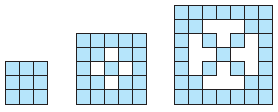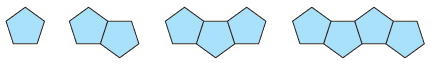# 4 Math for Elementary Education teachers problems

1) Look at the growing pattern below. How many squares will it take to make the nth figure in this pattern?2) What if we made a pattern by joining pentagons? If the length of each side of the pentagon is 3 units, then what would be the perimeter of nth pentagons joined together? Note: The perimeter is the distance around the outside of the figure.3) Variations of this problem have appeared in many places. Jack and Jill decide to start a rumor that there will be no school on the following Monday. What if each person tells exactly 2 people? How long will it take now? Assume that the rate for telling the next 2 people is 1 hour. That is, initially Jack and Jill know; after 1 hour, 2 more people know; after 2 hours, 4 more people know, . . . .

4) Consider the following table of numbers.

 x y 1 3 2 6 3 9 4 12 5 15 6 18 7 21

(a) Describe the pattern in your own words.

(b) Find the value of y when x is 100.

(c) Find a general expression for finding the nth term in your own words.

(d) Translate part (c) into function notation. f(x) =